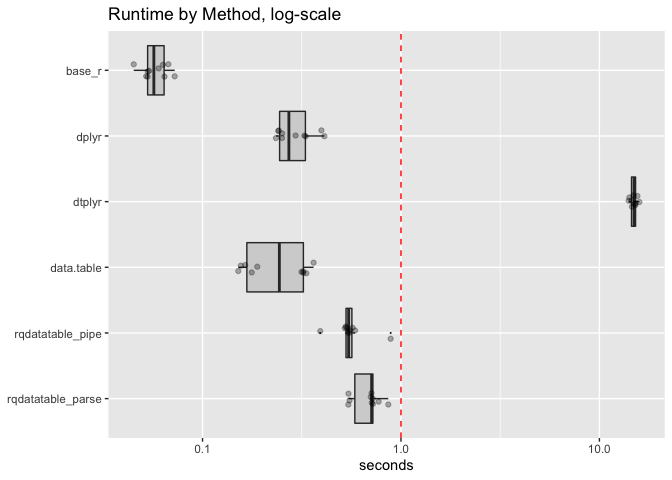# data.table is Much Better Than You Have Been Told

There is interest in converting relational query languages (that work both over SQL databases and on local data) into `data.table` commands, to take advantage of `data.table`‘s superior performance. Obviously if one wants to use `data.table` it is best to learn `data.table`. But if we want code that can run multiple places a translation layer may be in order.

In this note we look at how this translation is commonly done.

The `dtplyr` developers recently announced they are making changes to `dtplyr` to support two operation modes:

Note that there are two ways to use `dtplyr`:

• Eagerly [WIP]. When you use a dplyr verb directly on a data.table object, it
eagerly converts the dplyr code to data.table code, runs it, and returns a
new data.table. This is not very efficient because it can’t take advantage
of many of data.table’s best features.
• Lazily. In this form, trigged by using `lazy_dt()`, no computation is
performed until you explicitly request it with `as.data.table()`,
`as.data.frame()` or `as_tibble()`. This allows dtplyr to inspect the
full sequence of operations to figure out the best translation.

(reference, and recently completely deleted)

This is a bit confusing, but we can unroll it a bit.

• The first “eager” method is how `dplyr` (and later `dtplyr`) has always converted `dplyr` pipelines into `data.table` realizations.
It is odd to mark this as “WIP” (work in progress?), as this has been `dplyr`‘s strategy since the first released version of `dplyr` (verson 0.1.1 2014-01-29).
• The second “lazy” method is the proper way to call `data.table`. Our own `rqdatatable` package has been calling `data.table` this way for over a year (ref). It is very odd that `dplyr` didn’t use this good strategy for the `data.table` adaptor, as it is the strategy `dplyr` uses in its `SQL` adaptor.

Let’s take a look at the current published version of `dtplyr` (0.0.3) and how its eager evaluation works. Consider the following 4 trivial functions: that each add one to a `data.frame` column multiple times.

``````base_r_fn <- function(df) {
dt <- df
for(i in seq_len(nstep)) {
dt\$x1 <- dt\$x1 + 1
}
dt
}

dplyr_fn <- function(df) {
dt <- df
for(i in seq_len(nstep)) {
dt <- mutate(dt, x1 = x1 + 1)
}
dt
}

dtplyr_fn <- function(df) {
dt <- as.data.table(df)
for(i in seq_len(nstep)) {
dt <- mutate(dt, x1 = x1 + 1)
}
dt
}

data.table_fn <- function(df) {
dt <- as.data.table(df)
for(i in seq_len(nstep)) {
dt[, x1 := x1 + 1]
}
dt[]
}``````

`base_r_fn()` is idiomatic `R` code, `dplyr_fn()` is idiomatic `dplyr` code, `dtplyr_fn()` is a idiomatic `dplyr` code operating over a `data.table` object (hence using `dtplyr`), and `data.table_fn()` is idiomatic `data.table` code.

When we time running all of these functions operating on a 100000 row by 100 column data frame for 1000 steps we see each of them takes the following time to complete the task on average:

```        method mean_seconds
1:     base_r    0.8367011
2: data.table    1.5592681
3:      dplyr    2.6420171
4:     dtplyr  151.0217646
```

The “eager” `dtplyr` system is about 100 times slower than `data.table`. This trivial task is one of the few times that `data.table` isn’t by far the fastest implementation (in tasks involving grouped summaries, joins, and other non-trivial operations `data.table` typically has a large performance advantage, ref).

Here is the same data presented graphically.This is why we don’t consider “eager” the proper way to call `data.table`, it artificially makes `data.table` appear slow. This is the negative impression of `data.table` that the `dplyr`/`dtplyr` adaptors have been falsely giving `dplyr` users for the last five years. `dplyr` users either felt they were getting the performance of `data.table` through `dplyr` (if they didn’t check timings), or got a (false) negative impression of `data.table` (if they did check timings).

Details of the timings can be found here.

As we have said: the “don’t force so many extra copies” methodology has been in `rqdatable` for quite some time, and in fact works well. Some timings on a similar problem are shared here.Notice the two `rqdatatable` timings have some translation overhead. This is why using base `R` or `data.table` directly is, in general, going to be a superior methodology.

Categories: Opinion Statistics Tutorials

Tagged as:### jmount

Data Scientist and trainer at Win Vector LLC. One of the authors of Practical Data Science with R.